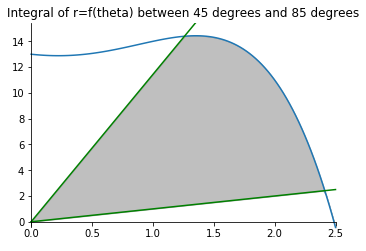Notebook

# Maths

## More on Integrals

The following chapter shows integrals applied to polar functions, also 3D figures produced by rotating (revolving) a 2D function and also line integration. The code used can be found here.

In :
% run ./maths_functions.py


### Polar Integration

In polar coordinate system, a point is defined by the angle $\theta$ at which it is relative to the y=0 axis, and the distance $r$ from the origin point.

The area of function $f({\theta},r)$ enclosed by limits $\theta_1$ and $\theta_2$ is $A=\int_{\theta_1}^{\theta_2}\frac{r^2}{2} d\theta$

The length of the function's line enclosed by those limits s $L=\int_{\theta_1}^{\theta_2} \sqrt{r^2 + (r')^2} d\theta$

In :
X = np.linspace(0,2.5, 100)
upper_degree = 85
bottom_degree = 45
def y_3(x):
return (-pow(x,4)+pow(x,3)+2*pow(x,2)-x+13)

In :
polar_integral_plot(X, y_3, bottom_degree, upper_degree)### Revolution

#### Volume

$V=\int_{A}^{B} \pi.(f(x))^2 dx$

#### Area

$A=\int_{A}^{B} 2.\pi.f(x).\sqrt{1+(f'(x))^2} dx$

### Line Integral

The integral of a smooth curve in a scalar or vectorial field is called line integral.

For a function $f(r(t))$ along a curve C, the integral is:

$\int_C f(r(t)) = \int_{t0}^{t1} f(r(t)).|r'(t)| dt$

If the curve is closed, the integral symbol can be shown as: $\oint_C f(x)$

A vectorial line integral should be broken down into components: $\int_C F ds = \int_C F_1.dx + F_2.dy + F_3.dz$

#### Solving using parametrization

Represent each variable as a function of $t$.

$x=x(t), y=y(t), z=z(t)$

Compute the derivatives dx, dy, dz. Find vector $\bar{r}(t) = (1-t).\bar{r_{start}} + t.\bar{r_{end}}$

Replace into equation for integral of f(r(t))

### Path Independence

If there is such $\phi$ exists, that satisfies:

$F=\cfrac{\partial \phi}{\partial x}.{\hat{i}} + \cfrac{\partial \phi}{\partial y}.{\hat{j}} + \cfrac{\partial \phi}{\partial z}.{\hat{k}}$

Then $\int_C F$ is path independent.